These series of function compute the shading factors on the different irradiance components, for unlimited PV sheds. PlantPredict supports various shading and incident angle modifier algorithms.

“3D” is the short hand way of referring to the depth pass stencil buffer shadow volume (DPSBSV) algorithm used for advanced shadow modeling in PlantPredict. It is carried out with the aid of the OpenGL API. The DPSBSV is one of the most common ways that shadows are calculated in video games today. It is a graphical solution, meaning the algorithm must first draw out all of the objects in a given DC field (i.e. all PV tables, as well as any external objects added which could cause shading), and after it has done so it will count up the number of pixels that are in shadow. This is carried out in a 5 step process.Shadow volumes are objects whose interior represents where the shadow is cast. This is done by projecting the vertices of a solar panel or object in the opposite direction to the sun position vector.Figure X: Objects without shadows2.) Render the solar panels3.) Render the shadow volume into the stencil buffer

The stencil buffer allows the user to make decisions on which areas to draw. Every stencil buffer pixel starts at 0; if a pixel sees the top of a shadow, the stencil buffer is incremented. If a pixel sees the bottom of a shadow, the stencil buffer is decremented. If it only sees a solar panel it is kept at 0.

4.) Redraw panels everywhere the stencil buffer is equal to 05.) Run occlusion test to get linear shading factor

So that it can perform the shading calculations as quickly as possible, the algorithm performs what is known as an occlusion test. This purpose of the test is to determine which hours have shading and to exclude any hours without shading from the calculation. Once an hour passes the occlusion test the linear shading factor can be determined simply by taking a ratio of the sum of the stencil buffer (shown in step 4) to the original field rendered with no shadows (shown in step 2).

For electrical shading the operation is a bit more complex. Instead of just taking a simple ratio of shaded area, the algorithm must determine which electrical strings are shaded. The number of total electrical strings in a DC Field is known based on the inputs, but In order to uniquely identify all electrical strings that are shaded the algorithm renders each one as a different color and passes these colors (represented numerically) into the stencil buffer. Finally, the algorithm counts up the total number of unique colors and divides it by the total number of electrical strings to yield the electrical shading factor for a given hour.

At this current point in time, the 3D shading algorithm is only calculating the shading factors for the direct beam component of irradiance. The impact on diffuse irradiance is still being calculated by PlantPredict’s original diffuse model which accounts for the impact of row-to-row shading and slope. The PlantPredict team is working to quantify an accurate impact of diffuse shading in the 3D model, and how it pertains to the addition of near field objects.

Linear –

The hourly linear shading factors are multiplied by the incident beam irradiance values so that a linear effective beam irradiance value can be determined.

Electrical –

The hourly electrical shading factors are multiplied by the incident beam irradiance values so that an electrical effective beam irradiance value can be determined.

## Inputs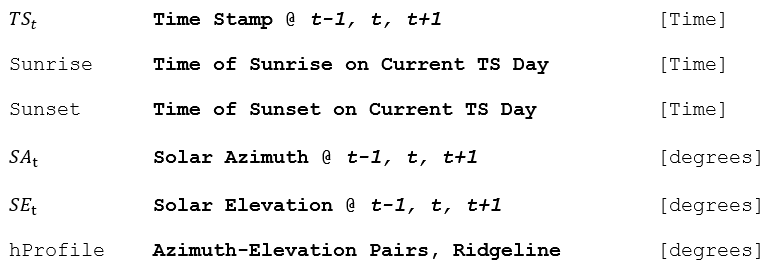## Outputs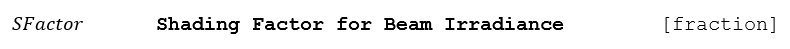## Algorithm Introduction

Satellite-derived irradiance data usually does not account for local topography. Horizon shading occurs when the beam component of the irradiance is occluded by a ridgeline, usually near the times of sunrise or sunset. The apparent sunrise and sunset times may be delayed and advanced, respectively, if mountains are present.

The shading effect is treated globally for the entire power plant, in other words, partial shading of a plant by distant mountains is not taken into account. This algorithm outputs a scaling value (between 0 and 1) that factors the direct beam irradiance proportional to the amount of time the sun was occluded during that simulation interval.

To estimate the amount of time the sun is blocked from far shading we use the solar geometry, the horizon profile (hProfile) and linear interpolation at 1-minute resolutions.

### The following summarizes the algorithm steps:

1.) Determine if the current timestamp could be subject to horizon shading

Using linear interpolation we can find the ridgeline elevation at any azimuth. Comparing these elevations with the sun’s elevations (at the same azimuth) over the course of the entire interval, we can determine if any horizon shading occurs.

The first step in this algorithm is to compile a list of the solar azimuth-elevation pairs (SEtSAt). If lowest solar elevation angle (has to be greater than 0) of the previous, current and next timestep is smaller than the highest elevation in the ridgeline profile then we will run the horizon shading analysis, otherwise we assume there is no shading.

2.) Estimate the time that the sun traverses the ridgeline

To determine if and when the sun traverses the ridgeline, we need to start with the timestamp that has the lowest solar elevation. The timestamps with the lowest solar elevation will always occur at the start (sunrise) or end (sunset) of a given interval. From the rotation of the earth, we know:

If 0 ≤ SAt ≤ 180 then the sun is rising and the lowest elevation is at the start of the interval, otherwise it’s at the end of the interval. For example, in the figure below, the sun is rising and the current timestamp is 7:30 AM, therefore the lowest elevation of the interval occurs at 7:00 AM. Sometime the lowest elevation of the interval is the Sunrise or Sunset time, this occurs when a sunrise or sunset event occurs during the current simulation interval.

With starting time, we can interpolate to find the solar azimuth and elevation at that time, and in turn the azimuth can be used to find the ridgeline elevation. While the ridgeline elevation is greater the solar elevation, the sun is still being obscured and we can step (interpolate) the next minute’s solar azimuth-elevation pair. Once we find the minute where the sun traverses the elevation profile, we store that time and break from the loop.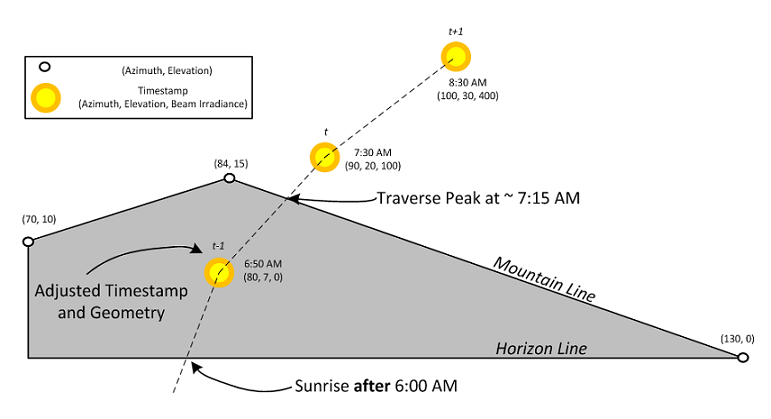### Figure 9. Example for Interpolating the Apparent Sunrise Time

3.) Estimate amount of beam irradiance that was obscured by the ridgeline

Now that we know the solar transit time above the ridgeline time, we need to calculate how much beam irradiance was blocked by the ridgeline. Consider the configuration diagramed in figure 9 above. Our current middle-of-irradiance interval timestamp is 7:30 AM and the transit time is 7:15 AM. If we found the transit time to be before 7:00 AM then we would have no shading to calculate, but in this case we know that the sun was obscured for 15 minutes of the current interval. We then estimate the amount of beam shading by finding what fraction of the interval was occluded, in this case 15 min / 60 min or 25%. This corresponds to a beam shading factor or SFactor of 0.72.

There are shortcomings of this algorithm, namely that it tends to overpredict beam shading because it treats the irradiance over every minute of interval as equal. In reality, the irradiance varies throughout the hour and therefor the shading factor should reflect this. This could be achieved in the future by interpolating the irradiances at t-1,t and t+1 to come up with a more accurate shading factor value.

Additionally, the algorithm could be improved by reducing the diffuse ground and diffuse sky irradiances but both of these changes need to be motivated by measured data before they can be implemented.

# Electrical Shading Models and Calculation

## Fractional Effect –

This model assumes that all module strings in a table are strung together according to vertical rank. Below, an example portrays a PV table that consists of 2 strings each consisting of 8 modules.The table is partially shaded on the bottom corner, and due to the fact that at least one module in the bottom string gets shaded the entire string experiences power loss due to electrical shading. The fractional effect value determines how much electrical shading power loss will be applied. A fractional effect of 100% will have the entire strings power go to zero once a portion (of greater than 1%) gets shaded. This will determine the electrical beam shading factor that gets calculated for the DC field and, in turn, the effective irradiance that the modules will see (explained further in Shade Factor Calculation).

A 75% fractional effect will reduce the entire string’s electrical beam shading factor to 25% of the linear beam shading factor, essentially reducing the loss due to electrical shading by 25%.

The below figure defines certain variables used in the Shade Factor (UshBES) calculation. Xm and Ym are the module’s length and width dimension, respectively. The other two dimensions, Xs and Ys represent the distance, length and width respectively, that the shadow overlaps the module.Knowing these dimensions at each time step, PlantPredict is able to calculate the shading factor using the following equations:In which Z is the shading response table (examples of two such tables were provided in the c-SI 3 Diode Default and Custom Shading Table sections above). Once the shading factor has been determined, PlantPredict will then use that to compute the Global Effective Beam Irradiance (Ee,ES) after it has been adjusted for electrical shading.UshBES,filt is a modified version of the shading factor values (UshBES) that were calculated earlier, but filtered to convert any value that is to 1 (no shading applied) if the area of the module shaded is less than 1%.

The next tasks that the logic performs is to recalculate the cell temperature to reflect the fact that the module is being subjected to a new Global Effective Beam Irradiance value. This calculation is done based on the Module Temperature model that is selected in the simulation settings. To see this calculation please refer to the Module Temperature section lower in this document.

Once Ee,ES and Tcell,mod are determined, the last step is for PlantPredict to plug these into the functions that compute the 5 parameters of the I-V curve and subsequently the single diode model so that the maximum power point energy after electrical shading (EMPP,ES) can be found.

The Electrical Loss % (as seen in the results screen) is calculated as follows: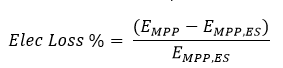In which EMPP is the maximum power point energy if it were to be calculated with linear shading.

This series of functions computes the shading factors on the direct beam component of irradiance. Given the sun’s azimuth angle and zenith angle (elevation angle’s complement), as well as the array’s orientation, spacing, tilt, and dimensions, the functions compute the geometry of the shadow cast by one row on an adjacent row as well as the fraction of the array that is shaded by tables within the array. Edge effects can be excluded based on user selection, which represents ignoring the unshaded edges of rows for DC fields that do not represent full arrays, typically used for the case when the array is designed for string inverters.

Figure 10 illustrates the direct beam row-to-row shading. The current tilt of the modules is represented here by 𝛽 for the fixed tilt or tracker tilt angle.### Figure 10. One-dimensional shed shading illustration of either a fixed-tilt or tracker, as viewed from the edge of the row

The following diagram, Figure 11, illustrates the variables used to calculate the direct beam shading on an array. In the figure, Δpp is the distance between tables, LT is the length of each table, and WT is the width of each table. Nr is the number of sheds. Although not shown, module azimuth angle, γ, is defined with due north being 0°, and is dependent upon user input DC field orientation. Also not shown, NT is the number of tables per shed. Although Figure 11 illustrates a fixed tilt array, all geometric definitions are the same for horizontal tracking arrays.### Figure 11. Two-dimensional mutual shed-on-shed shading. Example shows a fixed-tilt array with 180 azimuth (south-facing). Diagram adapted from: Dan Weinstock, Joseph Appelbaum, Shadow Variation on Photovoltaic Collectors in a Solar Field. Proceedings of the 23rd IEEE Convention of Electrical and Electronics Engineers in Israel, 2004.

Figure 12 shows the geometries that define a terrain slope. The angle of the slope is defined as 𝛼. This can be measured as the angle between the vector normal to the surface of the terrain and the zenith. The direction faced by the slope, called the aspect, is the azimuth from north of the vector normal to the surface of the terrain, γg.### Figure 12. Geometry of a slope. Terrain slope 𝛼 and aspect γg. Figure adapted and recreated from http://www.ces.iisc.ernet.in/grass/grass72/manuals/i.topo.corr.html

The diagram in Figure 13 illustrates the additional variables of slope 𝛼 and aspect γg used for modeling an array that is built along a slope. The module azimuth of the array points south, but the slope geometry is independent of the module azimuth.## InputsThe incidence angle is not used to compute the shading factor per se, but is a test to verify if the sun is behind the plane of the array.

## Outputs## Algorithm (Vector Method)

### Decomposition of a Slope

The terrain slope angle 𝛼 can be defined by the user for any aspect, or azimuth direction, γg. The aspect is defined according to the same convention as module azimuth angle.

To evaluate the shading that results from such a slope, it is helpful to decompose the slope into the two separate slopes that are along and perpendicular to the azimuth angle of the modules.

The component of the slope that is along the module azimuth angle affects the shading that occurs from one row onto another, or row-to-row shading. Since this shading occurs across rows, it is being termed Transverse shading. The contours of the slope in the transverse direction run parallel to the rows.

The component of the slope perpendicular to the module azimuth angle is along the rows of the array. It causes the tables of the rows to be at staggered heights, for a horizontal tracker system. This slope therefore creates shading between neighboring tables along a row, which is being termed Adjacent shading, or table-to-table shading. The overall shading impact of the slope 𝛼 is the shading due to both components. Depending on the relative location of a given table in the array, it might cast either Transverse shading, Adjacent shading, or a combination of the two.

The component of the slope that affects the Transverse shading between neighboring rows at a positive tilt is defined as transverse slope, 𝜓. Transverse shading is illustrated in Figure 14.

The component of the slope that affects the Adjacent shading between neighboring tables is defined as adjacent slope, 𝜙. For a tracker system, an adjacent slope facing away from the equator is considered positive. For a fixed tilt system, a westward facing adjacent slope is considered positive. Figure 15 illustrates the adjacent shading created between tables within a row at different heights along the slope.### Figure 14. Treatment of sheds on terrain with a transverse slope, 𝜓, showing the horizontal post-to-post spacing, Δpp. The figure could be interpreted as either: (a) the edge-view (looking east) of a northern-hemisphere fixed-tilt array on a north-facing slope at noon (sun due south at right), or alternatively: (b) looking north at a horizontal tracker on a west-facing slope at sunrise.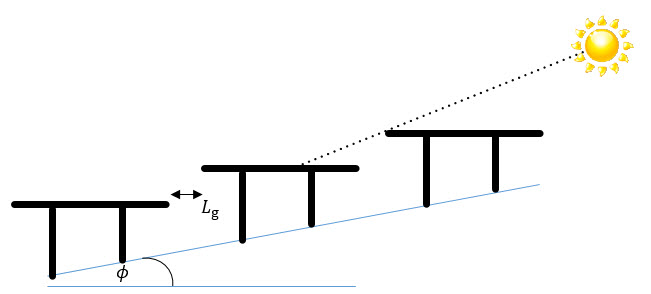### Figure 15. Treatment of sheds on terrain with an adjacent slope, 𝜙, showing the horizontal table-to-table spacing Lg of the slope angle 𝜓. The figure could be interpreted as either: (a) the front-view (looking east) of a northern-hemisphere horizontal tracker row on a north-facing slope at noon (sun due south at right), or alternatively: (b) looking north at a fixed tilt row on an west-facing slope at sunrise.

The components of the slope are decomposed into the perpendicular directions with the following calculations. The ground slope α can be positive or negative, while the aspect γg must be positive, from 0 to 2𝜋.

For a fixed tilt system with a positive latitude:For a fixed tilt system with a negative latitude or a tracker system with a positive latitude:For a tracker system with a negative latitude:The generalized shading fraction is calculated in the following algorithm.

1. First, define a right-handed table-centric Cartesian coordinate system for a 2-row geometry:

Origin:    Center of second table, at axis of rotation.

i:              positive in the direction of the first table (i.e. positive in the south direction for south-facing arrays).

j:              positive to the right along the lengthwise centerline of second table (from perspective of observer facing table).

k:             positive in the direction of the zenith.

The table causing shading in the row-to-row direction or the table-to-table direction (lengthwise along the rows) is the primary table, PTp or Prp, respectively, or Pp in general. The table that is shaded is the secondary table, Sp.Figure 16. Primary tables Pp relative to the shaded table Sp at a time other than solar noon. Primary table across the rows (or within the column), Prp, which creates Transverse shading. Primary table along (within) the row, PTp , which causes Adjacent (table-to-table) shading. The figure could be interpreted as either: (a) a N-S axis tracker system, or alternatively: (b) a south-facing fixed tilt system.

2. The solar unit vector s, transformed from spherical to the table-centric Cartesian coordinates, is:3. To calculate the shadow, we need to determine the vertical spacing between a corresponding point on the primary table (Pp) and the shaded table (Sp), conveniently determined by the height difference at the tops of corresponding posts of the tables. This spacing is defined as the glass-to-glass height difference Δgg.

4. The general equation of a plane is defined as a*x + b*y + c*z = 0. In our case, the tilted PV plane Sp intersects the plane normal to the surface of the earth at the y axis of our coordinate system, so the second row’s table sits in the plane a*x + c*z = 0, where a = sin(𝛽) and c = cos(𝛽). The equation for the plane in which the secondary table lies is therefore: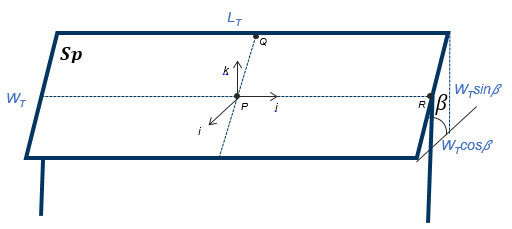### Figure 17. Detailed shaded table  geometry and coordinate system.

5. To find the percentage shaded as a function of the solar unit vector, first define the four vertices (the four corners) of the primary (Pp) table.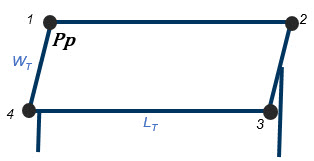### Figure 18. Vertex assignments of primary (Pp) table

The i,j,k coordinates of these vertices are given in the following matrix. The vertices are listed in the rows of the matrix, starting on the top-left of the table, and proceeding clockwise.6. Find the projection of the solar unit vector at each of these points Pp onto the plane containing the secondary table amounts to findthe intersection of the plane containing the secondary table with solar rays grazing each vertex of the primary table.### Figure 19. The projection of each of the points Prp along the solar unit vector s onto the plane containing the secondary table, where r is distance the solar ray has to travel between the two planes. This is at a time other than solar noon.

This can be evaluated as follows. The parameterization of the line made up of the solar ray passing through each vertex, n, as a function of r is given by:Here s is the solar unit vector. Substitute the formula for the line into the equation of the plane:Here r has become the distance the solar ray has to travel between the two tables. Determine the value of r, where a = sin 𝛽 and b = cos 𝛽: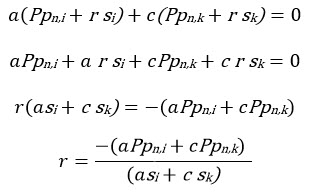Substitute r into the formula for the line to find the point of intersection in the secondary plane of the solar rays through each of the primary table’s vertices.7. Project the shading points Sp from 3D to 2D space, where y runs along the length of the table and x runs along the width of the table, as shown in Figure 20.### Figure 20. Relative locations of the shadow vertices P (shadow greyed) on the shaded table in 2D space.

In this coordinate system, y is simply the y-coordinate in the 3D panel-centric coordinate system, plus half the table length; x is the hypotenuse of i and k, added or subtracted from half the table width depending on the direction of i relative to the center of the table:8. If only one shadow falls on the table, it would be sufficient to determine the location of the corner of the shadow within the table to calculate the portion of the table that is shaded. However, if there might be multiple shadows on the table, the location of each shadow must be determined.

Next, find the location of the corners of PTn, the rectangular subset of the shadow that falls within the secondary table. The x-component of the shadow that exists on the secondary table is determined by testing the x-component of the first and third vertices of the shadow P against the x-component of the first and third vertices of the secondary table. The distance y is similarly determined with the same vertices. PTn can be calculated using either pair of opposite corners. It is assumed the planes of the tables are parallel to each other. The 2-dimensional coordinates of the secondary table’s vertices are given by V.9. The linear shading fraction SF of a single shadow on one table is then found by2: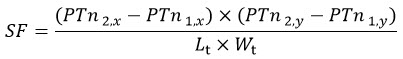Finding the shaded fraction could be implemented much more simply by using MATLAB’s rectangle intersection function Rectint, which requires as its arguments only the vertex vectors of polygons Sp and Pp.

10. For an entire array comprised of Nr rows or Nt columns (N in general) causing mutual shading, spaced equally, the shading fraction is slightly less because the first row or column remains unshaded, while the others are affected equally by their mutual shading.If multiple shadows from additional tables are considered, then μsh,B is calculated by a weighted average of the shading fraction on the relative number of tables.

For modeling portions of a larger array that has varying DC Field configurations, or when multiple small inverters and corresponding DC Fields make up an array, it may be preferred to assume that no unshaded columns- tables along the edge of the DC Field- exist. This is accomplished by assuming the Shading Factor applies to all tables in a row, regardless of proximity to the edge of the row, as though each row has an infinite number of tables. Proximity to the front of the array is still included, so the correct row count should be used. In order to consider the shading effects of surrounding DC fields and more accurately model shading losses for a DC Field as part of a larger array, this can be applied by activating Array-Based Shading in PlantPredict.

11. Reject all shading factors where the sun is found to be behind the plane of the array, i.e. the back of the module may be lit if the solar incidence angle is less than zero: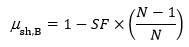The shading factor here is assigned to a value of -1 in order to distinguish it from 0 (fully shaded) and 1 (unshaded). A negative shading factor does not have any effect on the irradiance and will not contribute to the “shading loss” calculation.The shadows cast by surrounding tables are each determined independently based on their coordinate locations relative to the shaded table. Three cases exist: Transverse shading, which is caused by shading between tables across rows; Adjacent shading, which is caused by shading between tables within rows; and Diagonal shading, which is a combination of transverse and adjacent shading and is caused by tables located diagonally- neither within a row nor directly across rows. The formulas in the algorithm specific to each case are shown in the corresponding sections below identified by the relevant algorithm step.

The net shading fraction on the table is determined by evaluating the total area of the table covered by any of the shadows. This is calculated using an algorithm that determines the area affected by the shadows, accounting for the potential overlapping area of multiple shadows on the shared table.

The shadows considered in the current PlantPredict implementation are due to the tables shown in Figure 22 below, where each of the diagonal tables are in a dark or light red border.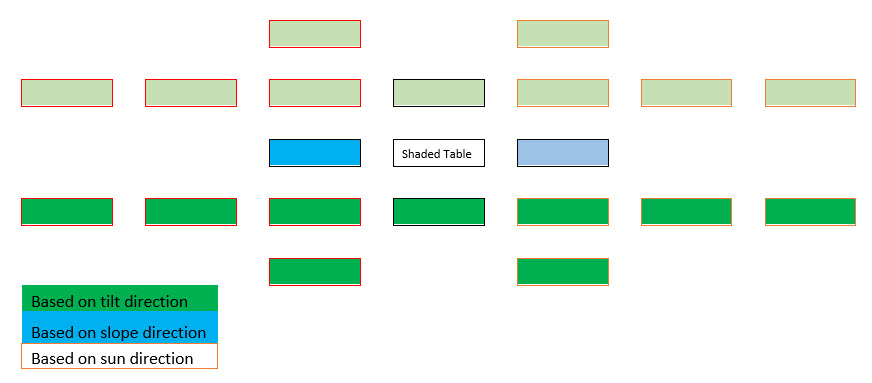### Figure 22. Tables from which shadows onto the shaded table are considered

Light green and dark green tables are considered depending on the tilt of the tables such that only the tables faced by the shaded table are considered. Light and dark blue tables are considered depending on the slope direction such that only the higher table is considered, since the lower table would never cast a shadow on front of the shaded table. Light and dark red-bordered tables are considered depending on the direction of the sun. These sets of tables are considered based on the sun azimuth angle relative to the azimuth angle faced by the tables.

To calculate the shadow, we need to know the vertical distance between the first row’s top edge and the second row’s top edge. This spacing is defined as the glass-to-glass height difference Δggrr and is a function of the post-to-post spacing Δpp and the slope of the ground in the row-to-row direction 𝜓.The tilt angle will always be positive for a fixed tilt system due to the user input restrictions. The primary row is always the one faced by the shaded row, which does not change for a fixed tilt system, but flips between rows (also known as columns) on either side of the shaded row, according to the tilt, for a tracker system. Therefore, for a tracker, the shadow of the table in the sun direction is considered based on the tilt by using the negative Δggrr for a negative tilt.

To calculate the shadow, we need to know the vertical distance between the first table’s top edge and the second table’s top edge, which can be calculated between any points along them since they are horizontal. This spacing is defined as the glass-to-glass height difference ΔggTT, and is a function of the table length LT, table-to-table spacing Lg, and the slope of the ground in the table-to-table direction Φ.Two adjustment factors, AdjFactor and bb1, are used to determine whether the primary table is in the positive or negative y-direction from the shaded table. This logic allows the algorithm to consider only the higher table along the row, which is the only one that can cast a shadow on the shaded table regardless of the sun direction.

For a tracker system,

AdjFactor = -1 and bb1 = 0, for slope Φ ≥ 0

For a fixed tilt system,

AdjFactor = -1 and bb1 = 0, for slope Φ < 0

Otherwise,

AdjFactor = 1 and bb1 = -1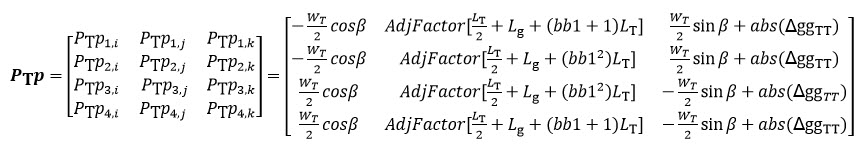The additional tables considered as casting shadows are located diagonally in the array from the shaded table (neither within a row nor within the column of table). The primary table coordinates Pp are found by a combination of the two coordinates used in Adjacent and Transverse shading. An example diagonal table Pp, in the first row and first column away from the shaded table, is shown below.It is only necessary to consider diagonal tables in the direction of the sun relative to the shaded table, since only those will cast their shadow onto that table. The algorithm determines whether the primary table is in the positive or negative y-direction from the shaded table, and the corresponding z-direction, by evaluating whether the azimuth of the sun, γs, is greater than the azimuth of the array, γ.

As shown in Step 8 in the general shading algorithm for finding the location of a shadow within the bounds of the shaded table, the location of overlap of two rectangles can be found. This methodology can be used to determine the area over which two shadows overlap each other based on the coordinates of the vertices of each shadow. Since only the total shaded area is important, the overlapping area must not be counted twice as shaded area.

To determine the total area shaded by an arbitrary number of shadows, an algorithm is devised to determine the overlap due to each permutation of the shadows. The total area is calculated as the sum of the overlap area calculated for each permutation made up of an odd number of shadows minus the sum of the overlap area calculated for each permutation made up of an even number of shadows.

Loss of irradiance due to shading is an important calculation for fixed tilt and tracker PV power plants. In addition to modeling direct beam shading, PlantPredict models diffuse shading. Diffuse shading is modeled using a diffuse sky-shading factor, which represents the effective fraction of sky dome lost due to near objects. These calculations are currently restricted to row-to-row shading.

The diffuse shading algorithm used by PlantPredict assumes a clear sky isotropic sky dome. It also assumes that rows of modules have infinite length. Diffuse shading is calculated for the midpoint of the table, represented by Point A in Figure 23, which is deemed representative for the entire table.## Inputs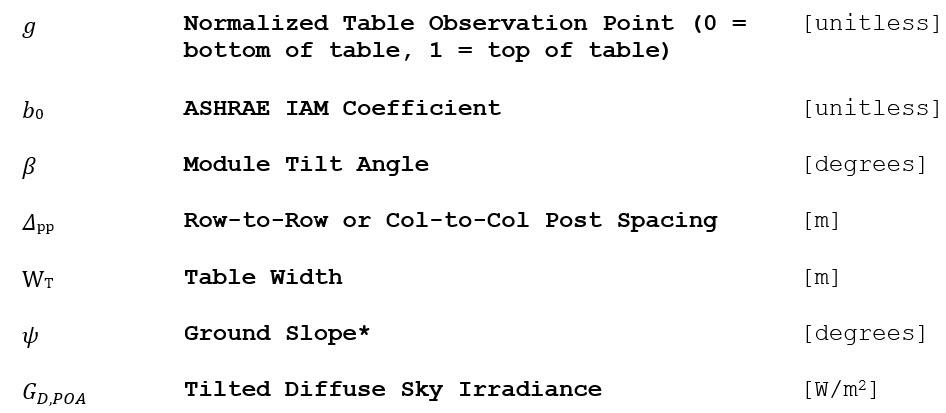*Found using equations from Near Direct Beam Shading

## Outputs## Algorithm

To estimate near diffuse shading factor, the portion of the sky dome visible to Point A is determined with and without the preceding row of modules. As seen in the equation below, the ratio of the former to the latter is the near diffuse shading factor (i.e. the unshaded fraction).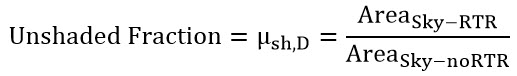AreaSky-RTR is the fraction of the sky visible to point A after row-to-row shading. It is not the area of the sky lost due to row-to-row shading. AreaSky-noRTR is the fraction of the sky dome that is visible to point A if the preceding row of modules was not present. Please note that, unless the module tilt = 0°, the denominator of the equation above is not equal to the full sky dome. This is because some of the sky dome is lost to the ground.  The geometry used to define both AreaSky-RTR and AreaSky-noRTR is shown in Figure 24.### Figure 24. Geometry definitions for diffuse shading model.

In Figure 24, β is equal to the module tilt. γ is the angle between the horizontal running through point A and the line connecting point A to point C (the top of the preceding row of modules). α is the angle between the surface normal from point A and the line connecting point A to point C.

Angles are defined as:The final equation for AreaSky-RTR is shown as:Where Atop = tan (β + γ).

Because of the periodicity of the tangent function, if α < 0 then AreaSky-RTR actually becomes the shaded area of the sky, as opposed to the visible portion of the sky, and the following adjustment is made to the output of the equation for AreaSky-RTR.The final equation for AreaSky-noRTR is nearly identical to that used for AreaSky-RTR and is included below.Where Aβ = tan (β).

Note that the equations above include the ASHRAE incidence angle modifier b0. PlantPredict uses the same equations to compute diffuse shading and diffuse incidence angle losses. When computing diffuse shading losses, b0 is assumed to be zero. When computing the diffuse incidence angle modifier, μD,IAM, the equation below is used.The effective sky diffuse irradiance on the tilted plane after diffuse IAM and shading, GD,Shd,IAM, is shown as:In the above equation, GD,POA is tilted diffuse sky irradiance.

For a sloped array, the function is changed such that γ is now a function of the ground slope.The diffuse shading algorithm accounts for ground slope that would place one row of modules higher, or lower, than the proceeding row. However, it does not currently account for ground slope along the length of rows. Examples of ground slope shading modeled by PlantPredict are shown in Figure 25 through Figure 27.

###Figure 25: Downward sloping ground, when shading is caused by the preceding row.### Figure 26: Downward sloping ground, when shading is caused by the subsequent row.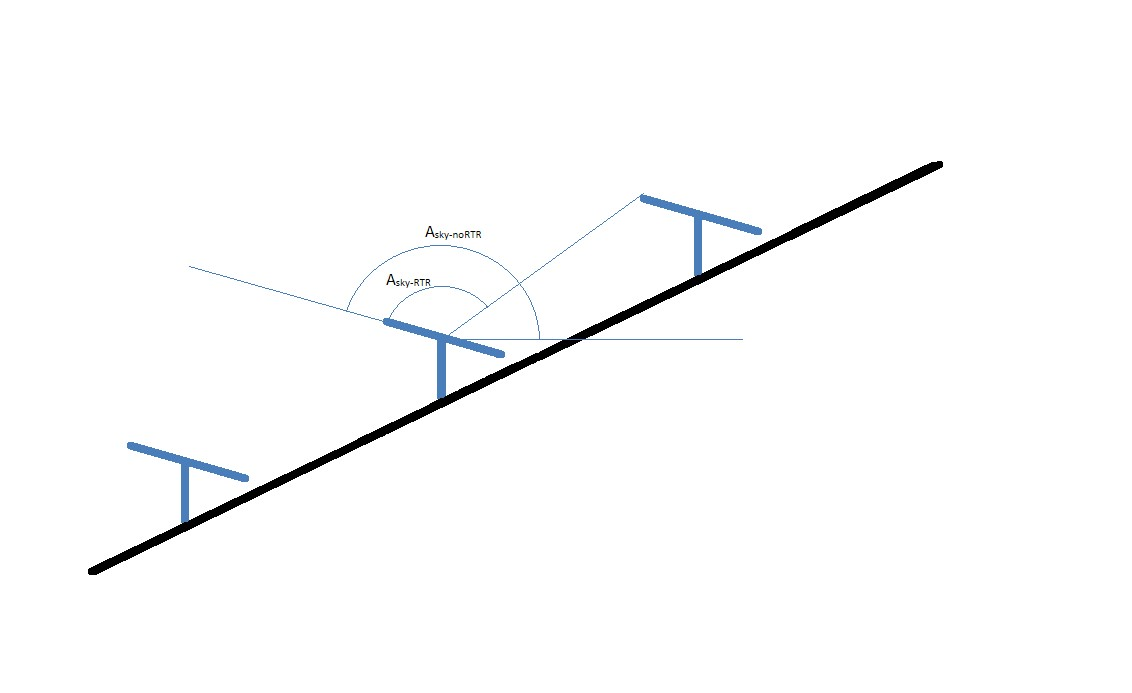### Figure 27: Upward sloping ground, when shading is caused by the preceding row.

This algorithm calculates the effective irradiance from the ground to the module corrected for incidence angle losses for a uniform array (tracker or fixed tilt).

## Algorithm

### Single Array

The irradiance from the ground onto a surface tilted with angle β, where GS,POA is the irradiance on the surface, is: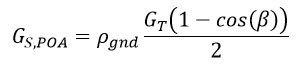When modeling the ground reflected irradiance on a utility scale solar array, the above equation is insufficient because it does not account for the IAM response of the module surface when receiving ground reflected irradiance. Also, it does not account for row-to-row “ground shading”. The subsequent sections adapt the above equation to account for IAM and ground shading effects.

### Regular Array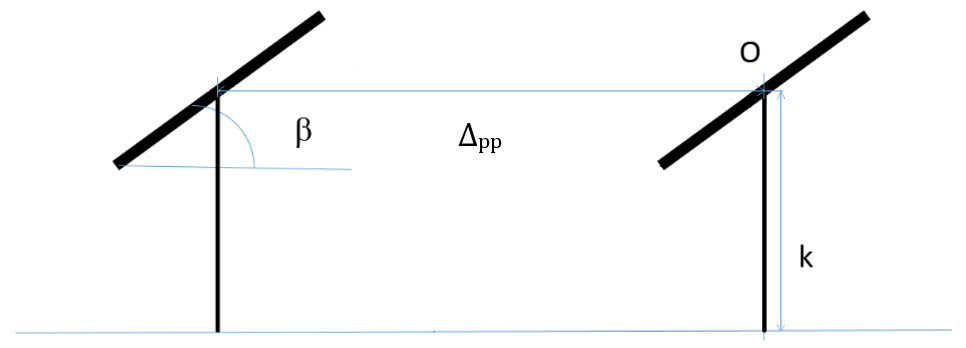### Figure 28. Geometry for diffuse ground shading calculation

Consider a two table cross section of a large array as shown in Figure 28. The total irradiance onto the segment between two tables is the sum of a diffuse component and a direct component. The diffuse component is found by calculating the sky view factor between the arrays. The direct component is found using the sun and array angles.  Rewriting the equation for the ground-reflected irradiance for a single plate to include both the diffuse and direct terms results in the following:Where:
Gs,POA-1D  is the irradiance reflected from the ground to the table surface.
GD,1D is the diffuse irradiance on the segment.
GB,1D  is the beam irradiance on the segment.

### GD, 1D, Diffuse

The total diffuse irradiance on a module in a regular array can be approximated by integrating the product of sky-view factor and IAM factor over a reasonable range in front of the module.We assume that the reasonable range is from a point on the row in front of the module (-Δpp where Δpp is row-to-row spacing) to the bottom field of view of the module (the closest point on the ground visible to the module), denoted by P, where P = -k cot β.### Figure 29. Angles and segments used to integrate the ground-view seen in a regular, repeating array.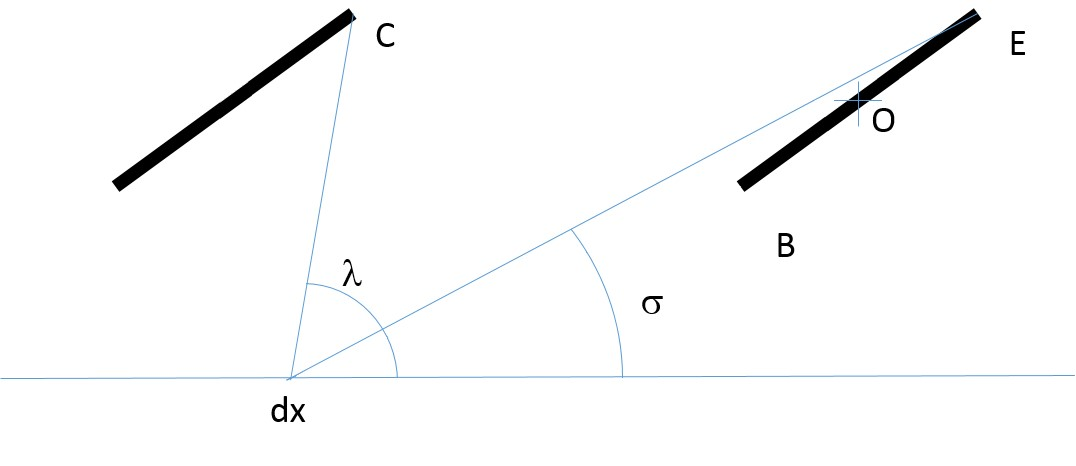### Figure 30. Additional angles and segments used in the geometric calculations.

In the figures above, point O is the origin. The coordinate system assumes that right and up are positive directions. Thus all values of x, are negative. Although not shown in the figures, WT is the table width (the distance between points E and B).  Below are the calculations of the geometry of the system:

The coordinates are: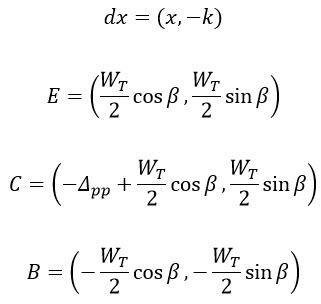And the angles are:If λ(x) < 0 then:The sky-view factor is then:The fractional sky-view factor is then:For simplicity, we are using the ASHRAE IAM model:Where the incidence angle,  is:### GB,1D, Direct

In this approximation, only the direct beam hitting the ground in front of the module is considered. The direct beam is a step function dependent on the sun angle with respect to the array. Consequently, the limits of integration are a function of solar and array position.